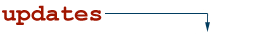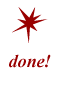# hexadecimalRefers to the base-16 number system, which consists of 16 unique symbols: the numbers 0 to 9 and the letters A to F. It is most commonly used online to assign color to Web pages. For example, the decimal number 15 is represented as F in the hexadecimal numbering system. The hexadecimal system is useful because it can represent every byte as two consecutive hexadecimal digits. It is easier for humans to read hexadecimal numbers than binary numbers. To convert a value from hexadecimal to binary, you merely translate each hexadecimal digit into its 4-bit binary equivalent. Hexadecimal numbers have either and 0x prefix or an h suffix. For example, the hexadecimal number: 0x3F7A translates to the following binary number: 0011 1111 0111 1010.

See also : Web designer
NetLingo Classification: Technical Terms